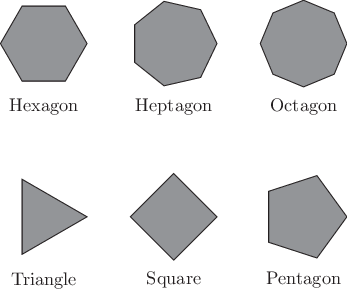# Pyxplot

## Examples - PolygonsDownload this example:

Using the polygon command

This example uses the polygon command, a rotation matrix, and nested for loops to construct diagrams of the first eight regular polygons.

### Script

```rotate(a) = matrix( [cos(a), -sin(a)], \
[sin(a),  cos(a)] )

subroutine makePolygon(Nsides, centre)
{
points = []
for i=0 to Nsides
{
call points.append(centre + \
rotate(i/Nsides*unit(rev)) * vector(1,0))
}
polygon points with fillcol gray50 col black
}

set nodisplay ; set multiplot

set texthalign center
set textvalign top

foreach datum x,y,Nsides,name in "--"
{
call makePolygon(Nsides,vector(x,y))
text name at x,y-1.25
}
0 0 3 Triangle
3 0 4 Square
6 0 5 Pentagon
0 4 6 Hexagon
3 4 7 Heptagon
6 4 8 Octagon
END

set display ; refresh

```